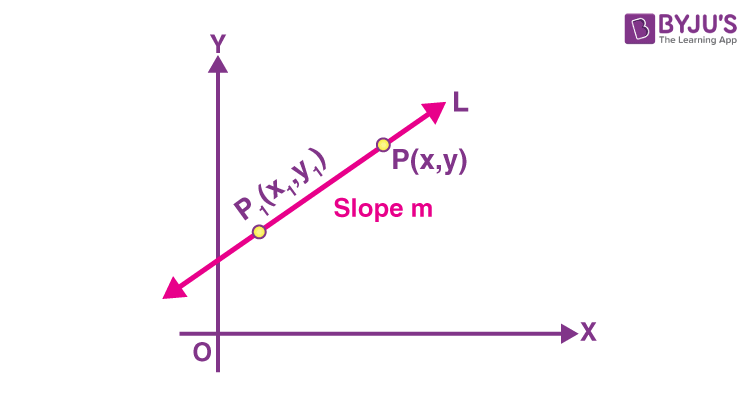Checkout JEE MAINS 2022 Question Paper Analysis : Checkout JEE MAINS 2022 Question Paper Analysis :

# Point Slope Form

In geometry, a line is a one-dimensional figure since it has length but no width. A line is made of a set of points that is extended infinitely in opposing directions. Two points in a two-dimensional plane determine it. However, there are different forms of equations of a line in a two-dimensional coordinate plane. The three mainly used methods are point-slope form, slope-intercept form, and general or standard form of the equation of a line. As the name suggests, the point-slope form involves the straight-line slope and a point on the line. It is possible to write the equations of infinite lines with a given slope, but we get a unique straight line when we specify that the line passes through a given point. Thus, only a point on the line and its slope is required to determine the equation of a straight line in the point-slope form.

## Point Slope Formula

The point slope form formula of equation of a straight line is given by:

y – y1 = m(x – x1)

Here,

m = Slope of the line

(x1, y1) = Point through which the given line passes

Let us derive the point slope form of a linear equation mathematically.

## Point Slope Form Derivation

Suppose that P1(x1, y1) is a fixed point on a non-vertical line L, whose slope is m.

Let P (x, y) be an arbitrary point on the line L.The slope of a line passing through the points (x1, y1) and (x, y) is the ratio of difference of y-coordinates and the difference of x-coordinates.

m = (y – y1)/ (x – x1)

⇒ y – y1 = m(x – x1)….(i)

As the point, P1(x1, y1) including all points (x, y) on L satisfies the equation (i), and no other point in the plane satisfies the above equation.

So, the equation (i) is undoubtedly the equation for the given line L.

Thus, the point (x, y) lies on the line with slope m through the fixed point (x1, y1), if and only if, its coordinates satisfy the equation.

y – y1 = m(x – x1)

Therefore, this is the point-slope form of a line equation.

Also, get the Point Slope Form Calculator and Slope Calculator here.

### Point Slope Form Examples

Example 1:

Find the equation of the line through (–4, 7) with slope -5.

Solution:

Given point is: (-4, 7)

Slope = m = -5

Let (-4, 7) = (x1, y1)

We know that the equation of a line in point slope form is:

y – y1 = m(x – x1)

Substituting the values,

y – 7 = -5[x – (-4)]

y – 7 = -5(x + 4)

y – 7 = -5x – 20

5x + y – 7 + 20 = 0

5x + y + 13 = 0

Hence, this is the required equation of the line.

Example 2:

Find the equation of a line passing through the points (1, –1) and (3, 5) in point slope form.

Solution:

Let the given points be:

(x1, y1) = (1, -1)

(x2, y2) = (3, 5)

Slope of the line passing through the given points is:

m = (y2 – y1)/ (x2 – x1)

= [5 – (-1)]/[3 – 1]

= 6/2

= 3

The equation of a line passing through the point (1, -1) with slope 3 is given as:

y – y1 = m(x – x1)

y – (-1) = 3(x – 1)

y + 1 = 3x – 3

-3x + y + 1 + 3 = 0

-3x + y + 4 = 0

Alternatively, the equation of a line passing through the point (3, 5) with slope 3 is given as:

y – 5 = 3(x – 3)

y – 5 = 3x – 9

-3x + y – 5 + 9 = 0

-3x + y + 4 = 0

Hence, the equation of the line in point slope form is -3x + y + 4 = 0.

## Frequently Asked Questions on Point Slope Form

### What is the point slope form equation?

The equation of a line passing through the point (x1, y1) having slope m is given by:
y – y1 = m(x – x1)
This is the point slope form equation.

### What is the point slope form equation of a line passing through the origin with slope m?

The equation of a line in point slope form is y – y1 = m(x – x1).
Given, (x1, y1) = (0, 0) and slope = m
Therefore, the equation of a line passing through the origin with slope m is: y – 0 = m(x = 0), i.e. y = mx.

### Is y = mx + b Point slope form?

No, the equation y = mx + b is the slope intercept form of the equation of a line with slope m and y-intercept b.

### How do you find the slope for two points?

Let (x1, y1) and (x2, y2) be the given two points, then the slope of the line passing through these points is:
m = (y2 – y1)/(x2 – x1)

### When can we use the point slope formula?

The formula of the point-slope form of an equation is used when we need to find the equation of a straight line given a point on it and the slope of the line.